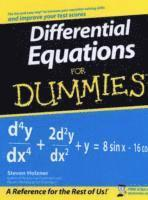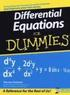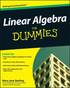Fler böcker inom
Format
Språk
Engelska
Antal sidor
368
Utgivningsdatum
2008-05-01
Upplaga
1
Förlag
John Wiley & Sons Inc
Illustratör/Fotograf
figures
Illustrationer
figures
Dimensioner
235 x 188 x 20 mm
Vikt
549 g
Antal komponenter
1
ISBN
9780470178140

### Du kanske gillar# Differential Equations For Dummies

(1 röst)
159
Skickas inom 7-10 vardagar.
Fri frakt inom Sverige över 159 kr för privatpersoner.
Finns även som
179
The fun and easy way to understand and solve complex equations Many of the fundamental laws of physics, chemistry, biology, and economics can be formulated as differential equations. This plain-English guide explores the many applications of this mathematical tool and shows how differential equations can help us understand the world around us. Differential Equations For Dummies is the perfect companion for a college differential equations course and is an ideal supplemental resource for other calculus classes as well as science and engineering courses. It offers step-by-step techniques, practical tips, numerous exercises, and clear, concise examples to help readers improve their differential equation-solving skills and boost their test scores.

## Passar bra ihop

1.2. +
3.De som köpt den här boken har ofta också köpt Linear Algebra For Dummies av Mary Jane Sterling (häftad).

## Kundrecensioner

Har du läst boken? Sätt ditt betyg »

## Övrig information

Steven Holzner is an award-winning author of science, math, and technical books. He got his training in differential equations at MIT and at Cornell University, where he got his PhD. He has been on the faculty at both MIT and Cornell University, and has written such bestsellers as Physics For Dummies and Physics Workbook For Dummies.

## Innehållsförteckning

Introduction. Part I: Focusing on First Order Differential Equations. Chapter 1: Welcome to the World of Differential Equations. Chapter 2: Looking at Linear First Order Differential Equations. Chapter 3: Sorting Out Separable First Order Differential Equations. Chapter 4: Exploring Exact First Order Differential Equations and Euler's Method. Part II: Surveying Second and Higher Order Differential Equations. Chapter 5: Examining Second Order Linear Homogeneous Differential Equations. Chapter 6: Studying Second Order Linear Nonhomogeneous Differential Equations. Chapter 7: Handling Higher Order Linear Homogeneous Differential Equations. Chapter 8: Taking On Higher Order Linear Nonhomogeneous Differential Equations. Part III: The Power Stuff: Advanced Techniques. Chapter 9: Getting Serious with Power Series and Ordinary Points. Chapter 10: Powering through Singular Points. Chapter 11: Working with Laplace Transforms. Chapter 12: Tackling Systems of First Order Linear Differential Equations. Chapter 13: Discovering Three Fail-Proof Numerical Methods. Part IV: The Part of Tens. Chapter 14: Ten Super-Helpful Online Differential Equation Tutorials. Chapter 15: Ten Really Cool Online Differential Equation Solving Tools. Index.